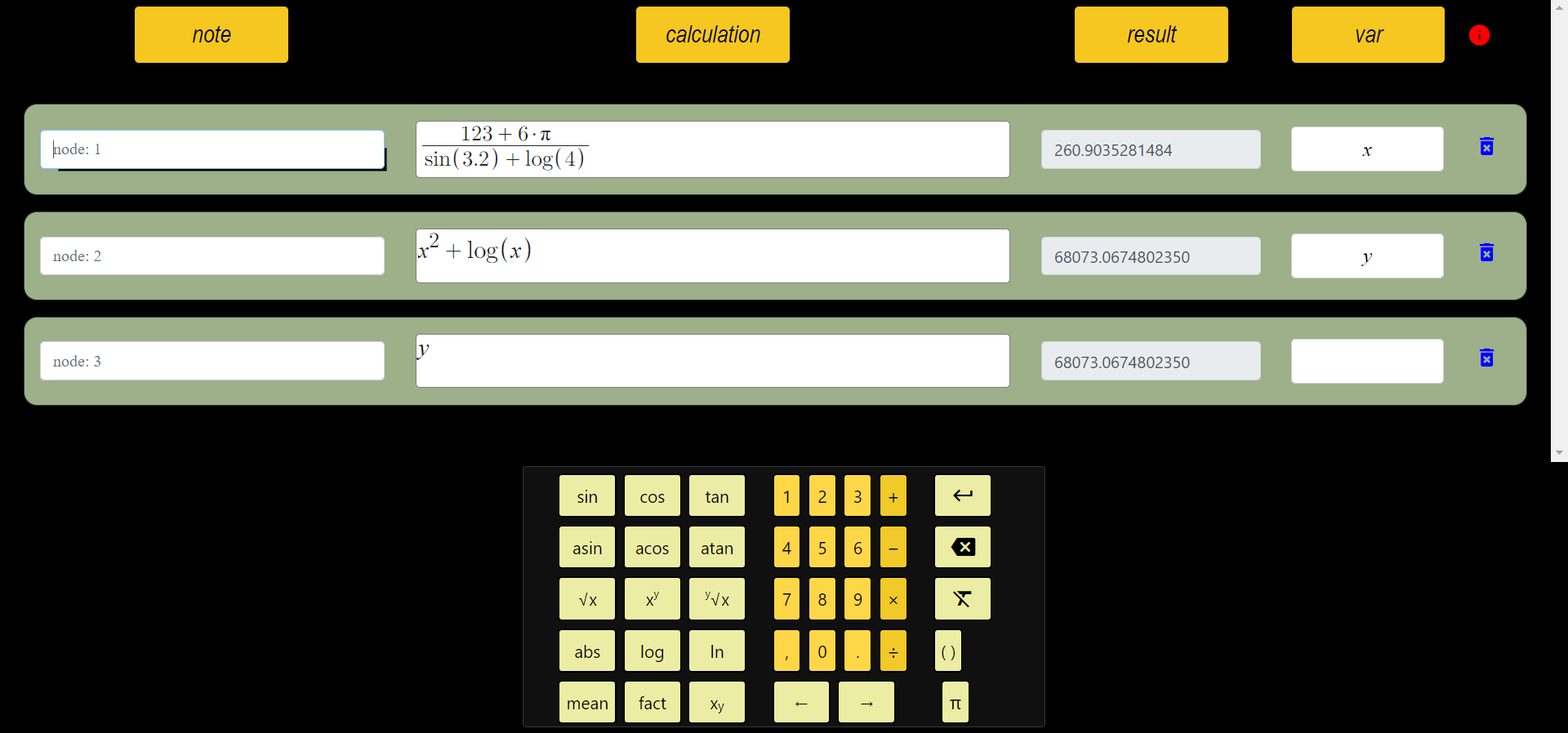# What is Scientific Calculator?

In general terms, a calculator is a tool that allows you to do arithmetic operations. A calculator can be a portable device, a computer software, even the abacus as we know it can be considered a calculator.

You can solve everyday problems with an ordinary calculator. However; chemistry, biology, physics, advanced mathematics, etc. In these matters, such calculators are sometimes insufficient. This is where scientific calculator comes into play. Advanced problems can be solved with functions such as logarithm, exponentiation, polynomial, and memory.

This article discusses the use of online scientific calculator in mathematics. This calculator is proving to be so important that it has become a necessity for students who are taking advanced mathematics courses. This is because these calculators have been designed with the intention of simplifying and shortening lengthy mathematical calculations.

A scientific calculator is a calculator that has an algebraic notation and can simplify expressions, perform calculus operations (such as integration and differentiation) as well as compute numerical approximations to transcendental functions. The first scientific calculators were developed in the 1960s. They were mainly mechanical or electromechanical devices with little memory capacity. The first all-electric scientific calculators were introduced in 1967, and they used vacuum tubes for logical operations and liquid crystal displays for display. The earliest known electronic scientific calculator was the Digitron, which was built by the Dixi company in 1964. It had ten constant functions, seven different memory registers with accumulator, built-in programmable square root function and three programmable keys.

## Calculator With Variables

Calculators with Variables are the best-sellers in the Scientific Calculator category. With more than 150 reviews and a 4.5 star rating, this calculator is a powerful tool for anyone in math class.

The scientific calculator with variables provide practical features like solving equations, simplifying fractions, and converting from fractions to decimals or percents. These calculators are also equipped with an LCD screen that displays scientific notation and other functions as well as hexadecimal conversions for memory storage purposes. They offer long battery life of up to three years when not in use and are designed to last through high school math courses or college calculus classes. An Introduction: Since calculators became a must-have item for classrooms across the world, they have been replaced by newer models

Tags: Online scientific calculator, variable calculator, scientific calculator, trigonometric calculator, solve math problem, functional calculator

###### All Articles

id: IfLspaXV6A